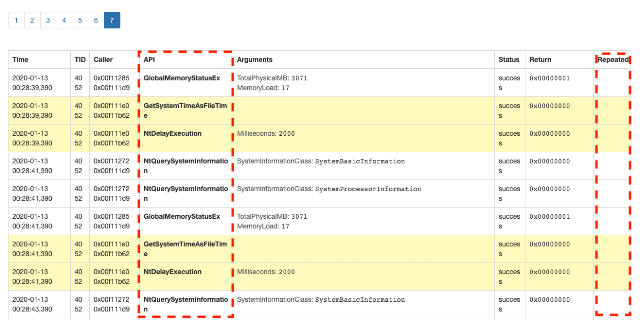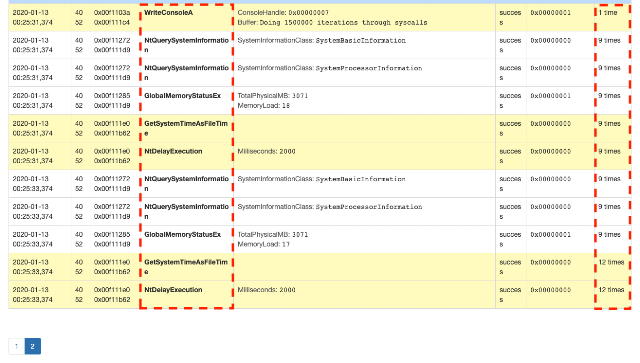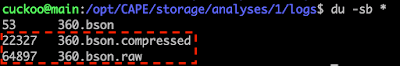## Wednesday, December 18, 2019

### Inline Loop Detection for Compressing API Call Traces

I have been working on a solution for compressing files containing trace of API calls coming out of a sandbox (Cuckoo sandbox ). This file holds events generated by a monitor module (DLL) which is injected into processes and hooks a pre-configured set of API calls. The main goal of this log is to describe interaction between processes and Operating System (Behavioural data).

This file can grow in size (memory demanding), complexity (CPU demanding) and noise (difficult to read) depending on two main factors:
• how long an analysed process is executed; and
• presence of specific control flow structures (e.g. loops).
This article proposes two methods for detecting looping patterns. The outcome of these methods is further used for compressing the above described sandbox log files without changing its structure.

Detecting loops by observing its execution is a complex task since not always a well defined pattern emerges. This article uses the terms "repeated element" and "loop body" to identify a content inside a loop statement. This element can be composed by a single call (repeated multiple times), multiple sequential calls and nested calls; its execution output can also be affected by control flow elements such as conditionals.

Repeated elements can also be affected by Pseudo-Random Number Generators. In this case, the final log presents more complex patterns. Algorithms described in this article do not consider this less common scenario and target detection of more general looping patterns.

.::[ Context and Example

Python-like pseudo-code snips are presented along this article for illustrating the proposed techniques. The following code snip describes a loop executing calls to functions labeled from 1 ("call_001") to 5 ("call_005"):

The body of the loop statement (line 2) executes functions "002" and "0035 times and function "004" every time "i" is even. The behavioural log produced by executing the script above would be:

Each entry in this log contains a position (line number "line[x]") and an identifier ("c_00[x]") to a call. A new entry is added to this file every time a function is executed. Taking a closer look, it is possible to observe a repetition pattern located between lines 2 and 14. In this specific example, these lines describes the execution of a loop and could be compressed to the following form:

The compressed output above has the following properties:
• log size was reduced in 3 times (66% compression rate);
• preserved the original order that functions are executed; and
• it is easier to read;
Next sessions describe ideas to reach the compression exemplified above. The first idea uses Suffix Trees  and Non-Overlapping Longest Repeated Subset  and the second uses N-Gram .

.::[ Method 001: Non-Overlapping Longest Repeated Substring

The first idea consists in using Non-Overlapping Longest Repeated Substring  which is a well stablished algorithm with complexity equal to O(n^2). This algorithm can be applied to our specific problem for finding repeated subsets of entries which represent repeated calls (and possible loop). Each entry in the log file must be mapped to a checksum and this value should represent a setup to a API call (position, parameters, function name etc).

The following pseudo-code shows a simplified representation of this algorithm.

The first part of this code (lines 1 to 4) creates a vector named "data" containing checksums calculated from each log entry (we call these checksums "primitive" tokens). After that, the algorithm runs in a loop until "calculate_lrs()" returns an empty value. "replace_lrs()" function replaces the all instances of LRS (Longest Repeated Substring) vector within "data" with a token. This token and its respective LRS vector are saved in a translation map (dictionary named "map"). Next step is reducing repeated tokens and count how many times it repeats (line 14). At the end, tokens are translated back to vectors by using the translation map (line 15).

The second "while" listed in line 10 detects repetition patterns inside LRS vectors (this creates recursion). Next code snip shows a use case for this scenario.

In this case the vector "[2, 2]" would be replaced with a unique token in the original data vector and the main loop moves to its next iteration using this transformed vector.

The final output for the input vector above would be "[(0, 2), (1, 2), (2, 8)]". The first element of each set is a primitive token and the second element is the amount of repetitions.

The code below is a Python implementation of the logic described above.

The output to the code above is:

Log entries (line 1) were compressed (line 2) and the information of repetition was appended. This algorithm could be applied in a real scenario for compressing behavioural logs in a very efficient way in terms of compression but not really in terms of computational resources usage. During our experiments with real world behavioural logs, we could detect two critical drawbacks of this approach:
• memory consumption - as all log entries have to be loaded in memory in order to run the "find_repeated_substring()" function; and
• processing time - the complexity is not that bad O(n^2) but the algorithm is called many times and this impacts the overall efficiency of the script.
This method can be used in a scenario with many small/medium size log files when maximum efficiency compression is a hard-requirement.

These limitations pushed this research to experiment other approaches. Since the log files are relatively big and loops usually perform small and concise block of operations so the full coverage (to all sizes of looping body) is not really necessary.

.::[ Method 002: N-Gram

N-Gram is a contiguous sequence of "n" items from a given sample . This concept can be used for representing repeated patterns and could be used in a solution to the problem investigated in this article.

Since human-generated loop contents are limited in size, the solution proposed in this section can be bounded according to a parameter. This parameter is named "Compressor Level" in this article and holds a value (positive Integer) representing the maximum size to a loop body (repeated pattern). This determines how many pattern detectors the algorithm will create with size varying from 1 to "Compressor Level".

The following pseudo-code shows the idea described in this section:

This algorithm first pre-initialise a list of compressors (this component will be better explained later) using the above mentioned "Compressor Level" parameter. This "for" loop (line 2) creates multiple compressors containing N-Grams with "N" ranging from 1 to a positive integer passed as parameter ("i" on line 3).

The loop listed from lines 5 to 10 connects Compressors. Elements are added to the smallest Compressor and its output is added to the next one in line with it. This cycle repeats until the algorithm reaches the last Compressor.

A buffer in a compressor acts like a queue (first in first out) of size equal to "level" times 2. This buffer is incremented and if buffer is full an analysis is executed. This analysis simply checks if the first half of buffer is the same as the second. If the answer to this check is positive then the buffer is compressed eliminating the repetition.

The following code is a Python implementation of the method described in this section.

The next snip shows the output of the code above.

This approach is able to detect loop patterns and compresses them dynamically (inline) without using big chunks of memory. This algorithm could be implemented in a solution for compressing behavioural logs and optimising resources usage in a Sandbox.

.::[ Conclusions

The algorithm described in this article can be used for identifying repetition patterns in any kind of data series. We successfully used it for compressing behavioural log files coming out from a Sandbox used for analysing malware. The main benefits of using the algorithm described in this paper were:
• Logs got compressed;
• Defeated malware strategies to DOS this kind of analysis;
• Behavioural report became easier to read;
• Since the amount of data reduced, searches got faster;
• Saved memory and disk space; and
• It became easier to visually identify loops;
Next step is to move this code inside Cuckoo Sandbox  and check how effective it compresses "tasks" structures (this is how Cuckoo calls each sandboxing session) before importing them into Mongo just by compressing behavioural logs (of all processes).

Here goes some screenshots from our preliminary tests using Cuckoo Sandbox CAPE . We developed this small PE sample  which runs a loop going through few calls just for testing our approach:Figure 01: Number of pages of API call entries BEFORE compressionFigure 02: Number of pages ofAPI call entries AFTER compressionFigure 03: Behavioural analysis report section BEFORE compressionFigure 04: Behavioural analysis report section AFTER compressionFigure 05: Compressed BSON sizes in disk
The final BSONs (compressed JSON) got 3 times smaller after compressed this impacts the load of Mongo, size of final JSON report and speed of searches using the UI.